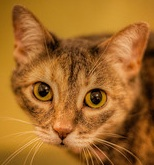# Machine Learning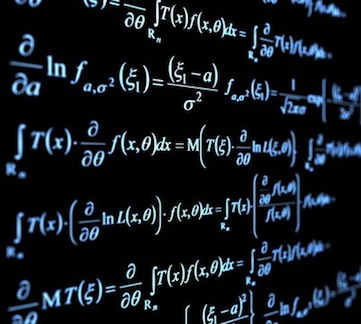# Machine Learning

## Examples

• face detection
• spam filtering
• recommendation systems
• character recognition
traditional computing: Hand-chosen conditionals and params.
ML algorithms: Rules are learned from data

# Training

• Learn rules by training on labelled data
• same algorithm, different data
• generic = power

# Let's try something

## The problem

Should I bother?...
Does it have any cat pics????

# Cat Detection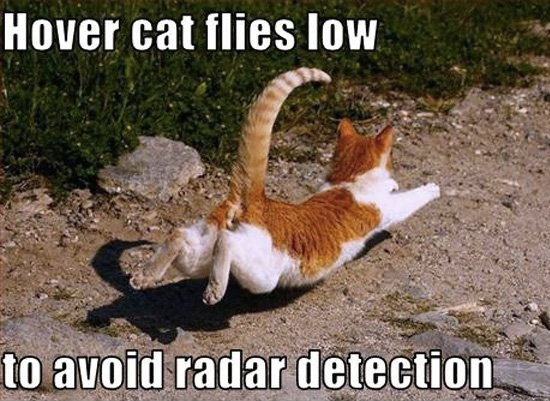## Breaking it down

does this image contain a cat?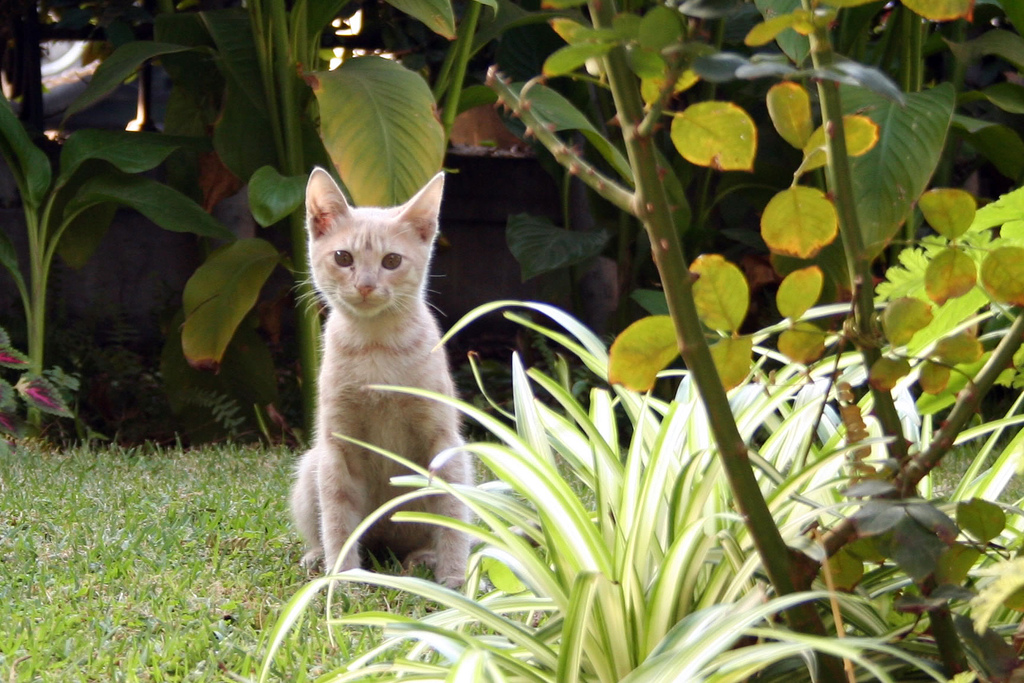## Breaking it down

is this subsection a cat head?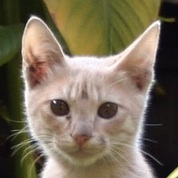## We need a classifier

• Takes a piece of data, tells you which class it's in.
• Trained with known classifications
• Bayesian (spam), k-nearest neighbors, support vector machines, neural networks.

## neural networks

```      ```
function runNetwork(input) {
var net = {"layers":[{"0":{},"1":{}},{"0":{"bias":5.1244897557632765,"weights":{"0":-3.591317000303657,"1":-3.594502936141513}},"1":{"bias":1.4480619514263766,"weights":{"0":-5.021099423700753,"1":-5.055736046304716}},"2":{"bias":0.655017127607016,"weights":{"0":-3.9842614825641096,"1":-4.020357237374914}}},{"0":{"bias":-3.093322979654723,"weights":{"0":7.328941033927063,"1":-5.699647431673055,"2":-3.879799253666414}}}]};

for (var i = 1; i < net.layers.length; i++) {
var layer = net.layers[i];
var output = {};

for (var id in layer) {
var node = layer[id];

var sum = node.bias;
for (var iid in node.weights) {
sum += node.weights[iid] * input[iid];
}
output[id] = (1 / (1 + Math.exp(-sum)));
}
input = output;
}
return output;
}
```
```
Not going to go into how it works, but these floating point values are trained. Optimization problem, try to find floating point values that minimize the error in the training set.

## input and output

```    ```
net.train([
{ input: [0.7, 0.1, 0.3], output:  },
{ input: [1.0, 0.8, 0.7], output:  },
{ input: [0.5, 0.6, 0.7], output:  }
]);

var output = net.run([0.5, 0.5, 0.6]);   // [0.001]
```
```
• output is `` if cat, `` if not
• input is `[?, ?, ?, ...]`

## What is the input?

Pixels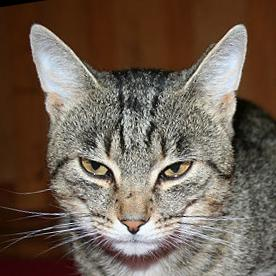too much variation
Edgesleaves shape information
want to make it easy on the network, giving it data it can get a better handle on, data that doesn't vary too much

• Captures strength + direction of edges
• Pixels → array of #s from `0` to `1`
• perfect!
• `48x48` canvas → HOG of length `1176`

## Collection

• negatives: 1000s of cat-free crops
• positives: 1000s of cat head crops
• resize to `48x48`
• node-canvas

## Training

```    ```
var hog = require("hog-descriptor");
var brain = require("brain");

var data = pics.map(function(pic) {
return {
input: hog.extractHOG(pic.canvas),
expected: [pic.cat ? 1 : 0]
}
})

var net = new brain.NeuralNetwork();

net.train(data);
```
```

### Is this subsection a cat head?

```    ```
function isCat(canvas) {
var features = hog.extractHOG(canvas);

var prob = net.run(features);

return prob > 0.9;
}
```
```

### Does this image contain a cat?

• Test "windows" at different scales, locations
• Combine overlapping detections
• Weed out spurious detections

# JavaScript?

## Not As Fun

• Weak typing / implicit conversions
• `NaN`...`0/0` or `undefined + 7`?
• worse: `Math.abs(null) = 0` silent destruction
• Speed